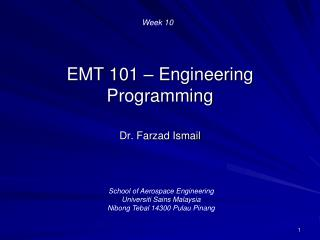DownloadDownload PresentationEMT 101 – Engineering Programming

# EMT 101 – Engineering Programming

Download Presentation## EMT 101 – Engineering Programming

- - - - - - - - - - - - - - - - - - - - - - - - - - - E N D - - - - - - - - - - - - - - - - - - - - - - - - - - -
##### Presentation Transcript

1. Week 10 EMT 101 – Engineering Programming Dr. Farzad Ismail School of Aerospace Engineering UniversitiSains Malaysia NibongTebal 14300 Pulau Pinang

2. What is Matlab? • C++ is a programming language but Matlab is software which are used for many engineering applications • Matlab also has its own programming language • Matlab has its own interpreter, no need to compile or link source codes • Data types are not declared–MATLAB: automatically assigns the appropriate type when you initialize a variable

3. Matrix Laboratory? • MATrixLABoratory • MATLAB performs matrix (or vector) manipulations and computations • Output to screens are automatic (no need cout) • Input to code-use input • Type help input to learn more

4. Vectors and Matrices • Elements in an array can be assessed using indices • Example: b = [ 2 5 6 9 10 12 15 22] • Type b(2) in MATLAB will give you 5 • b(4) gives you 9 • Type b(2:4) is an array [ 5 6 9] • b(3:end) gives you [6 9 10 12 15 22]

5. Matrices (2D Array) • Given a 3 x 3 matrix A = [ 3 4 5; 8 6 2; 7 9 11] • A(1,1) is 3 • A(1,3) is 5 • A(2,:) is a 1D (1 by 3) array of [8 6 2] • A(:,2) is a 1D (3 by 1) array of [4; 6; 9]

6. Operators • sum(x); max(x), min(x), mean(x) • sum of matrices or vectors • vector multiplication:dot product vs. vector product • always be careful with the operators * and .* • a*b is totally different than a.*b • matrix multiplication; matrix products • transpose of a matrix: use ’

7. Linear Algebra Example • Solving a system of linear equations: Ax = b • matrix vector multiplications • two ways of solving a system of equations: • (i) x=inv(A)*b or (ii) x=A\b See Tutorial exercise 2

8. Control Structures • If-else statements (similar to C++) • The format for switch case: Note that <value> cannot be a range like in the if branch. This is a limitation of case • switch <variableName> case <value1> statement ... case <value2> statement ... otherwise statement end

9. For Loop in Matlab • Example with the dot product operations of vectors a and b of sizes n = 1000 sum = 0 for i = 1:1:1000 sum = sum + a(i)*b(i) end

10. Tutorial Construct a vector of with range with increment of 0.1. Solution: In Matlab, this can be done by setting X= [-1:0.1:1] Do-It-Yourself-Exercise • Determine another array • Next, determine the derivative • Plot the functions using plot(X,Y1,XY2)

11. Tutorial Solution %copy this code into the Matlab Script Editor. X=[-1:0.1:1]; %by including a “semicolon”, the output will %not be shown in the Command Window Y1=X.^2; %notice the used of “.^” instead of only “^” Y2=gradient(Y1); %command to find the gradient plot(X,Y1,X,Y2) %command to plot a graph legend('Y1','Y2') %command for the graph’s label

12. Exercise 1 • Find the slope of a function F=cos x * exp(2x)* x^3 and plot the slope between [0, 3]. • Plot both the slope and the function F on the same plot.

13. Exercise 2 • Using subroutines, write a MATLAB program to solve • an arbitrary matrix problem A= M*N where M and N are matrices in which you need to input the numbers on your screen. Note M and N has a size mxm. • Finding an inverse of the matrix